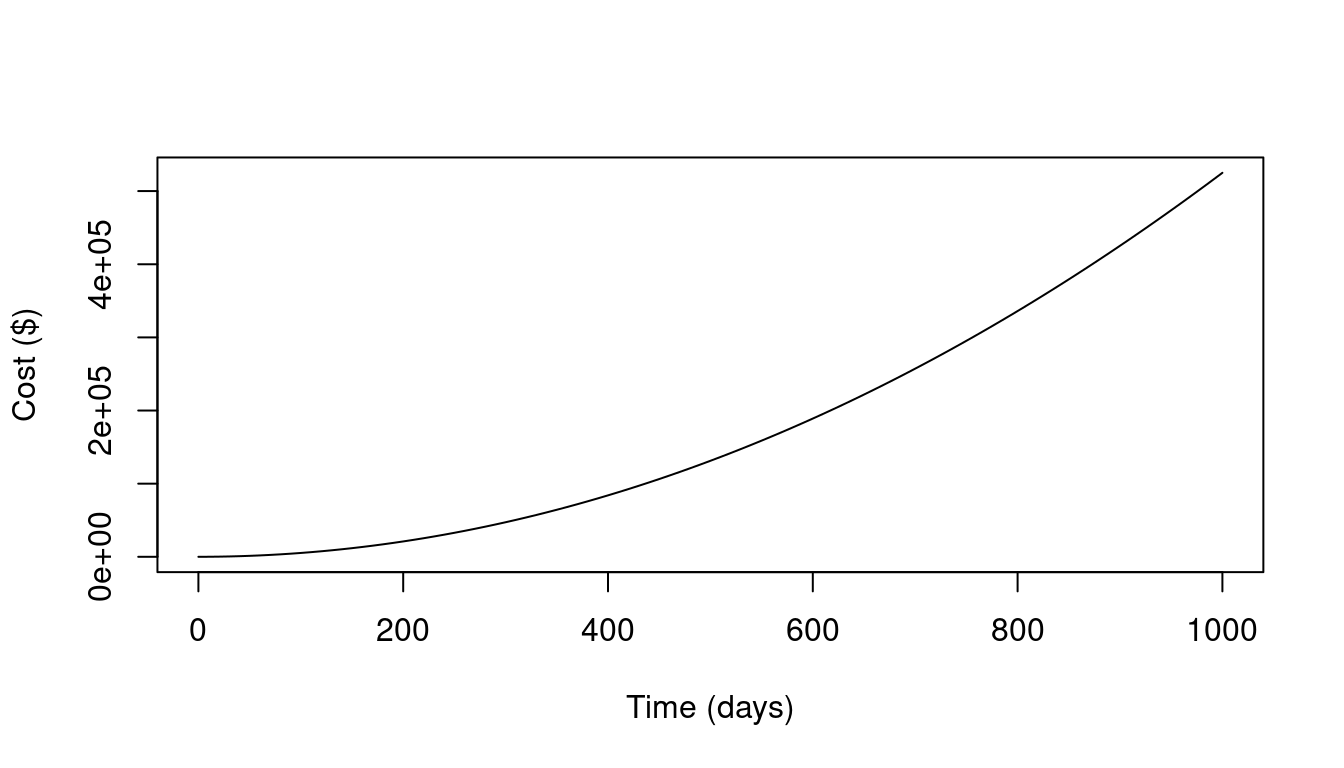The main point of the optistock package is to produce cost-per-fish (CPF) curves for determining how expensive it is to stock fish on any given day based on the management outcome. The model combines information from a species growth and natural mortality, as we well as hatchery rearing costs to determine the economically optimum time to stock fish that will result in the lowest cost.

A simple CPF curve might look something like the following: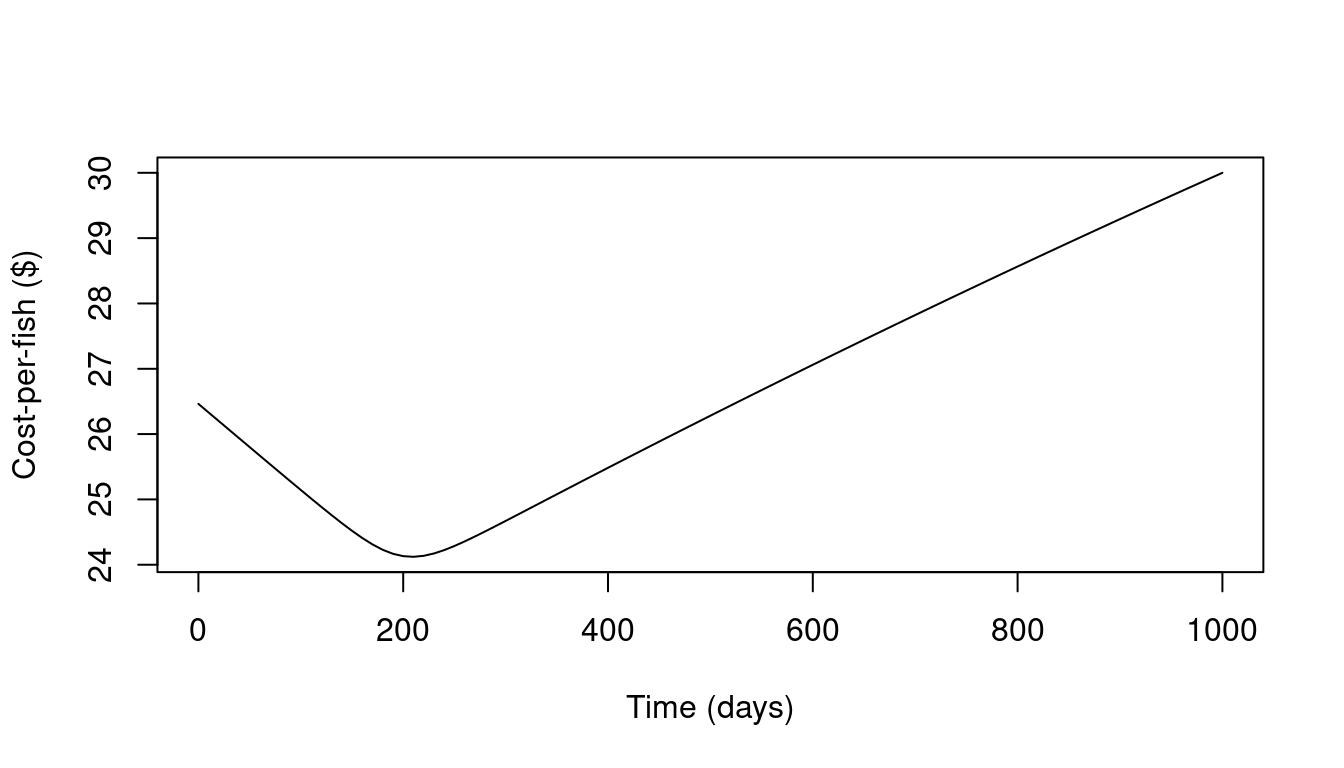The code to produce the above curve is called with a single function that includes arguments for mortality and cost curves. Growth is used in the model to determine how long it will take for fish to get to a certain length. Here is the code that produced the above curve. While it may look unwieldy at first it is actually fairly concise once all the compoents are understood.

curve(
cost_per_fish(
time_at_stocking = x,
time_at_rec = 1000,
n_recruits_desired = 200,
cost_fun = linear_total_cost,
cost_fun_args = list(int = 20, beta = 0.01),
mort_fun = exp_mort,
mort_fun_args = list(
m_init = 0.001, m_inf = 0.0001, alpha = 0.05, t_scale = 200
)
),
0, 1000,
xlab = "Time (days)", ylab = "Cost-per-fish ($)" ) ### Shiny App You can create your own examples by calling optistock_app(), which will open a shiny application that allows you to adjust the model. ## Package Details This package contains all the necessary functions to be able to compute cost-per-fish for different fish stocking scenarios so long as growth, mortality, and hatchery costs are known (or assumed). The model starts with a management objective of having a certain number of fish of a certain length in a waterbody at some point in the future. Starting there one can work backwards using a growth function to determine how long (on average) it will take fish to grow that long. Once the time-to-length is calculated a mortality function can be used to determine how many fish need to be stocked at any given time to result in the desired number of fish of length $$L$$ at recruitment time $$t_R$$. ## Growth functions The growth functions in this package are used to determine how long it will take a fish to reach length $$L$$. There are both growth functions and inverse growth functions. Growth functions take an input of time $$t$$ and corresponding growth parameters (i.e. for von Bertalanffy – $$L_{\infty}$$, $$k$$, and $$t_0$$). The returned value is the length $$L$$ at time $$t$$. Inverse growth functions take the same growth parameters as the corresponding growth function, but take a length $$L$$ and return the time $$t$$ that the average fish will reach that length. ### Growth Curve time <- 1:1000 linf <- 50 k <- 0.4 / 365 t0 <- -0.5 * 365 len_at_age <- vbgf(time, linf, k, t0) plot(len_at_age ~ time, type = "l", xlab = "Age (days)", ylab = "Length")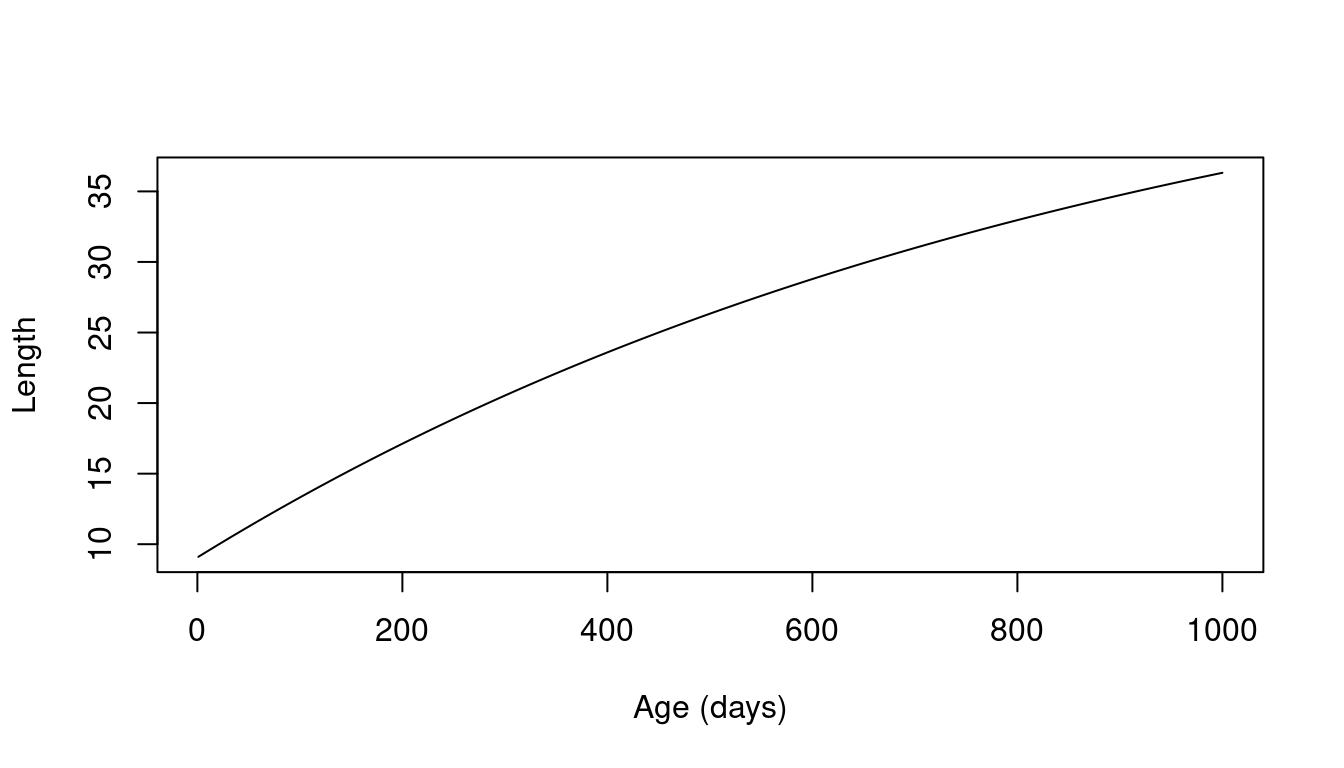### Inverse Growth Curve age_at_len <- inv_vb(len_at_age, linf, k, t0) plot(age_at_len ~ len_at_age, type = "l", xlab = "Length", ylab = "Age (days)")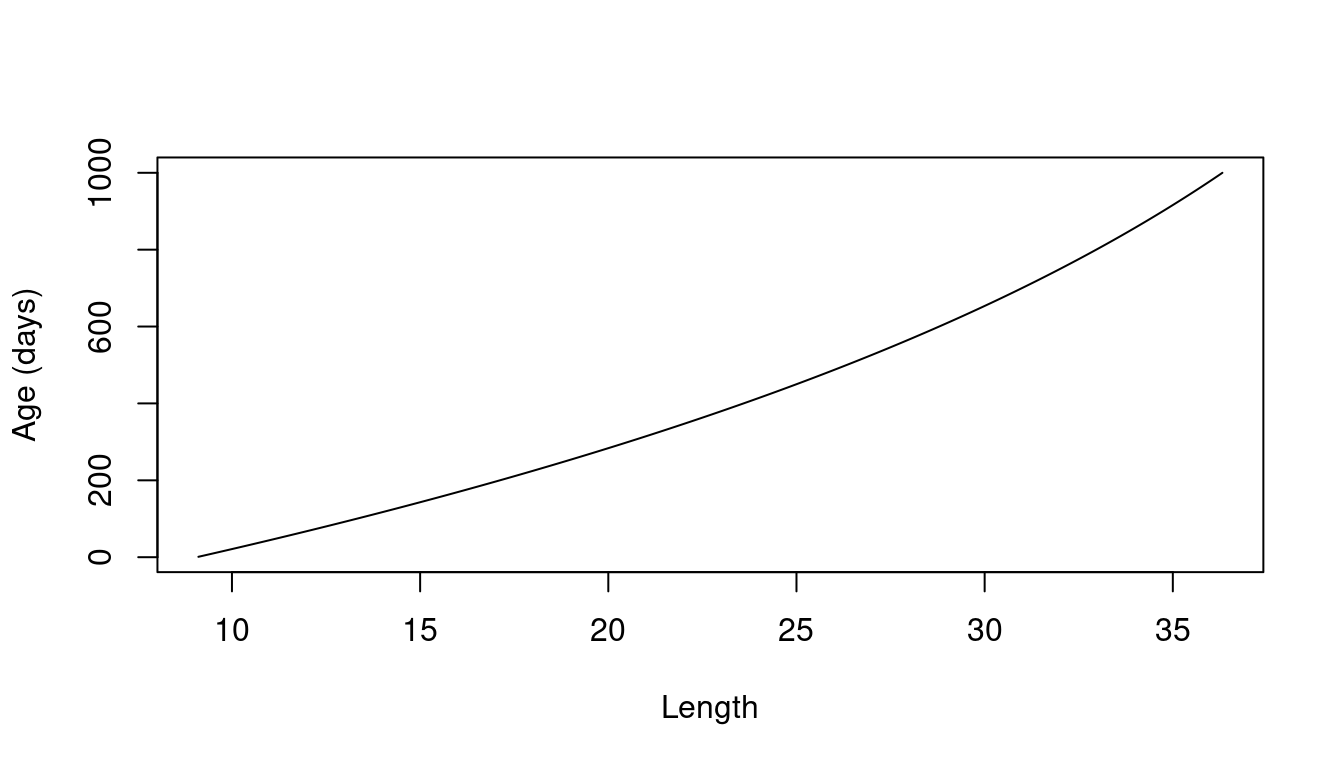## Mortality functions There are currently 8 different mortality functions in optistock. These return the natural mortality at time $$t$$ given the input parameters. curve( exp_mort(x, m_init = 0.5, m_inf = 0.05, alpha = 0.05, t_scale = 200), 0, 1000, xlab = "Time (days)", ylab = "M" )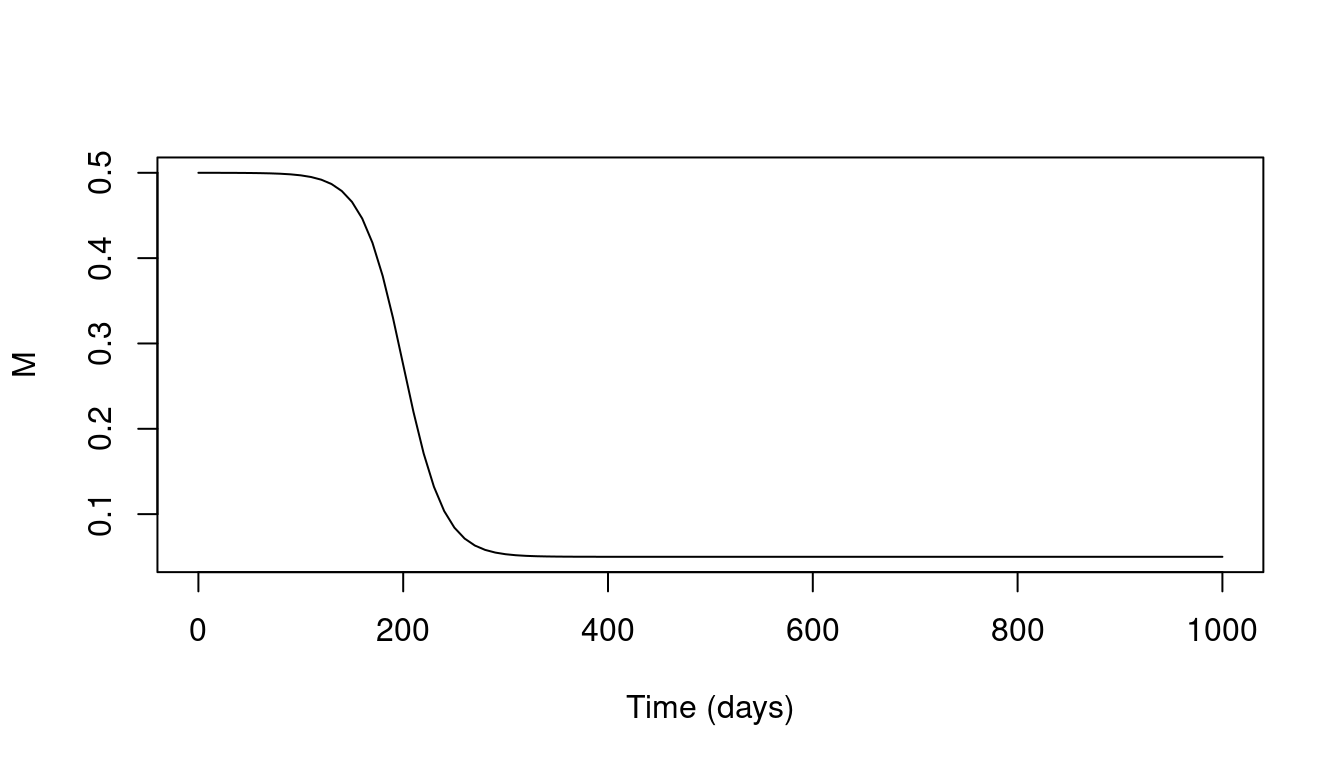curve( decreasing_mort(x, m_init = 0.5, m_inf = 0.05, alpha = 0.99), 0, 1000, xlab = "Time (days)", ylab = "M" )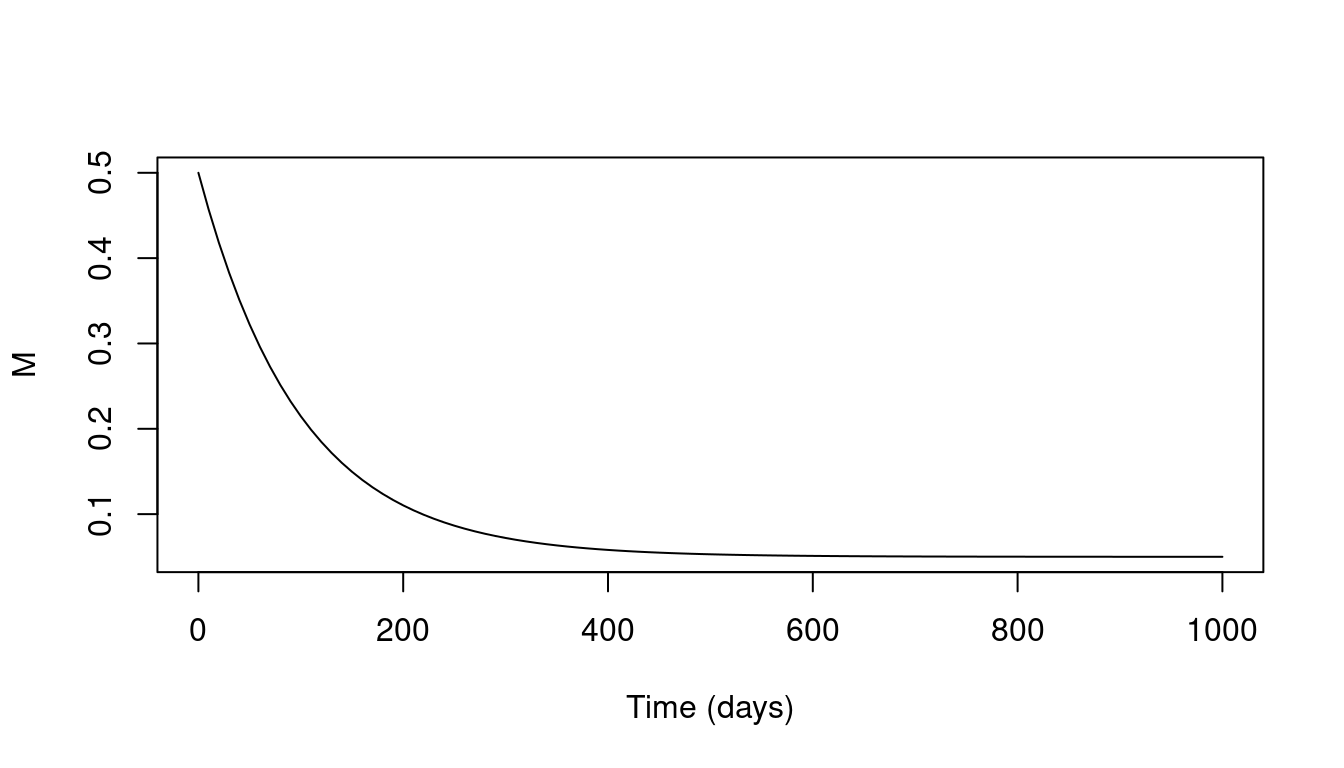## Cost functions There are two types of cost functions available to calculate total costs to raise fish to a certain day. One is a linearly increasing function where total costs increase at a constant rate across time. The other is an exponentially increasing function where the total cost increases at a greater rate as time goes along. In both cases the cost can be made to increase exponentially along with the number of recruits raise if that behavior is so desired. ### Linear total cost recruits <- 1 int <- 1.2 beta <- 0.05 curve( linear_total_cost(x, recruits, int, beta), 0, 1000, xlab = "Time (days)", ylab = "Cost ($)"
)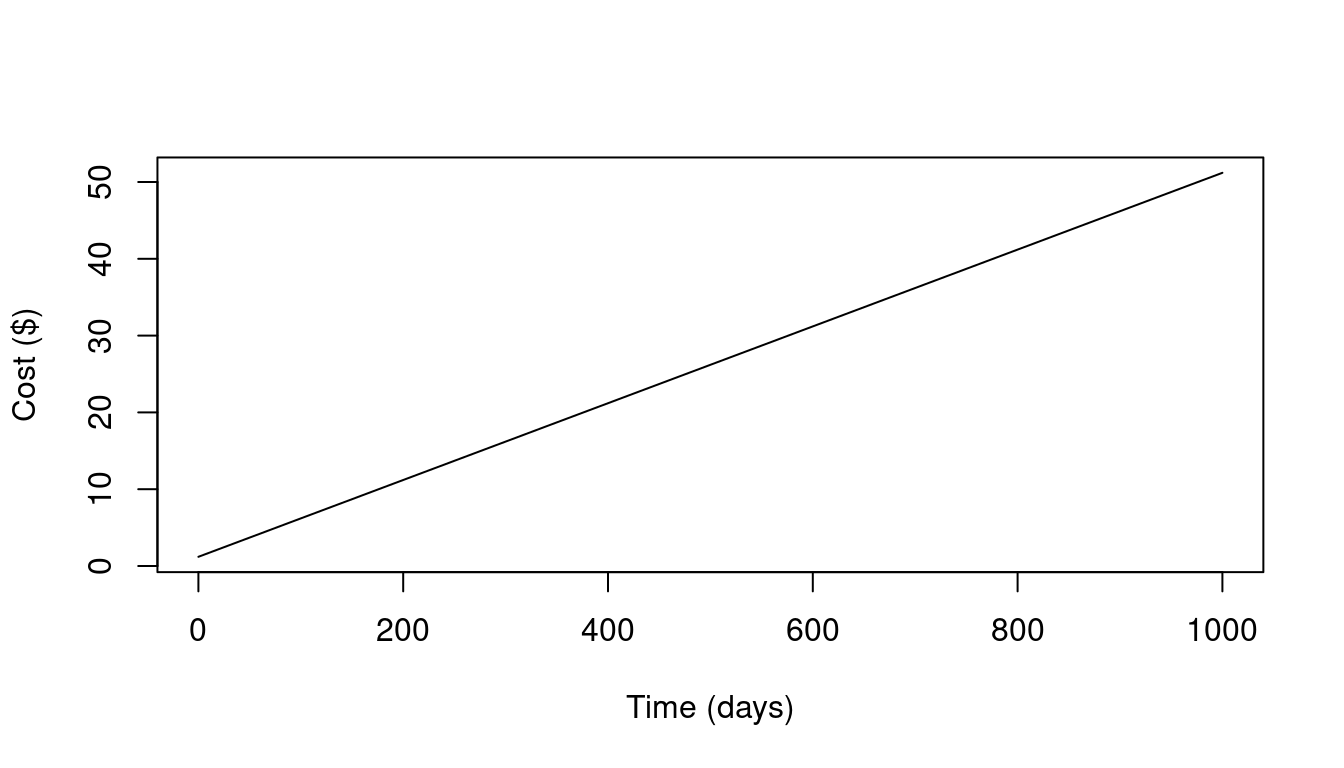### Exponential Total Cost

init_cost <- 0.01
time_slope <- 0.01
time_exp <- 1.05
rec_slope <- 1
rec_exp <- 1
curve(
total_daily_cost(x, recruits, init_cost, time_slope, time_exp, rec_slope, rec_exp),
0, 1000,
xlab = "Time (days)", ylab = "Cost (\$)"
)